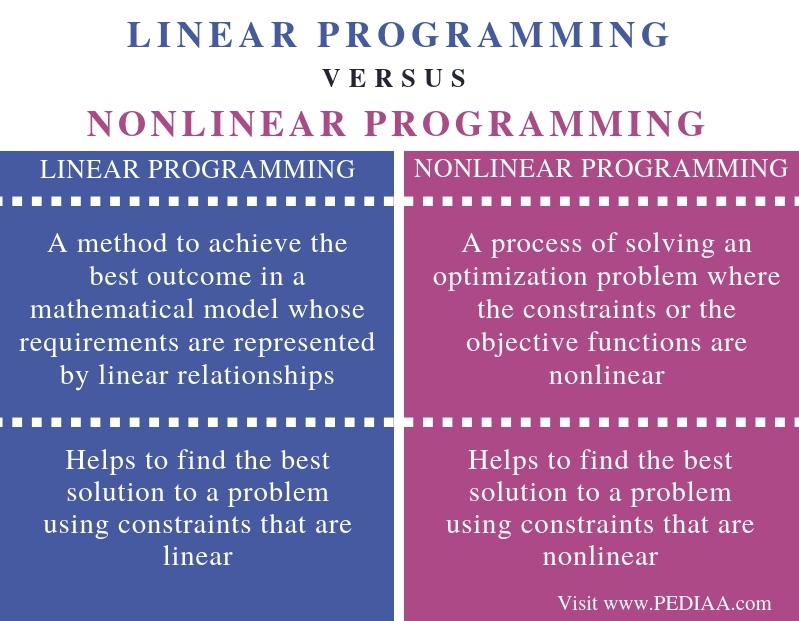# What is the Difference Between Linear and Nonlinear Programming

The main difference between linear and nonlinear programming is that a linear programming helps to find the best solution from a set of parameters or requirements that have a linear relationship while a nonlinear programming helps to find the best solution from a set of parameters or requirements that have a nonlinear relationship.

Linear programming is an important concept in optimization techniques in mathematics as it helps to find the most optimized solution to a given problem. On the other hand, nonlinear programming is the mathematical method of finding the optimized solution by considering constraints or objective functions that are nonlinear.

### Key Areas Covered

1. What is Linear Programming
– Definition, Functionality
2. What is Nonlinear Programming
– Definition, Functionality
3. What is the Difference Between Linear and Nonlinear Programming
– Comparison of Key Differences

### Key Terms

Linear Programming, Nonlinear Programming## What is Linear Programming

Linear programming is a mathematical method that allows determining the best possible outcome or solution from a given set of parameters or a set of requirements. These parameters or requirements have a linear relationship. Moreover, the main advantage of linear programming is that it helps to perform modeling or simulation to find the best solutions according to the available money, energy, resources, time, space and other related factors or variables. Furthermore, the outcome of linear programming is maximizing profit and reducing cost.Figure 1: Example Graph for Linear Programming

The basic components of linear programming are as follows.

Decision variables – Quantities to determine

Objective Function – Describes how each decision variable affect the property that should be optimized

Constraints – Represents how each decision variable would use limited amounts of resources

Data – Explains the relationships between the objective function and the constraints

## What is Nonlinear Programming

Nonlinear programming is the process of solving optimization problems that concern some of the nonlinear constraints or nonlinear objective functions. It involves minimizing or maximizing a nonlinear objective function subject to bound constraints, linear constraints, nonlinear constraints, etc. These constraints can be inequalities or equalities. In addition, nonlinear programming helps in analyzing design tradeoffs, selecting optimal designs, computing optimal trajectories and portfolio optimization and model calibration in computation finance.Figure 2: Example Graph for Nonlinear Programming

There are two types of nonlinear programming as follows.

### Unconstrained Nonlinear Programming

Unconstrained nonlinear programming involves finding a vector x that is a local minimum to the nonlinear scalar function f(x). Quasi-Newton, Nelder Mead, and Trust-region are some common unconstrained nonlinear programming algorithms.

### Constrained Nonlinear Programming

Constrained nonlinear programming involves finding a vector x that minimizes a nonlinear function f(x) subject to one or more constraints. Interior-point, sequential quadratic programming, and trust region reflective are some common constrained nonlinear programming algorithms.

## Difference Between Linear and Nonlinear Programming

### Definition

Linear programming is a method to achieve the best outcome in a mathematical model whose requirements are represented by linear relationships whereas nonlinear programming is a process of solving an optimization problem where the constraints or the objective functions are nonlinear. Thus, this is the main difference between linear and nonlinear programming.

### Usage

Moreover, linear programming helps to find the best solution to a problem using constraints that are linear while nonlinear programming helps to find the best solution to a problem using constraints that are nonlinear.

### Conclusion

The main difference between linear and nonlinear programming is that the linear programming helps to find the best solution from a set of parameters or requirements that have a linear relationship while the nonlinear programming helps to find the best solution from a set of parameters or requirements that have a nonlinear relationship.

##### Reference:

1. “What Is Linear Programming (LP)? – Definition from Techopedia.” Techopedia.com, Available here.
2. “Nonlinear Programming.” Wikipedia, Wikimedia Foundation, 26 Oct. 2018, Available here.

##### Image Courtesy:

1. “Linear programming example graph” By en:User:Jacj – Own work by en:User:Jacj (Public Domain) via Commons Wikimedia
2. “Nonlinear programming” By Krishnavedala – Own work (CC0) via Commons Wikimedia